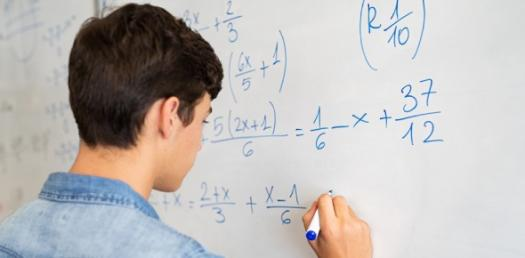# Jss1 Mathematics

32 Questions | Attempts: 6628Settings.

• 1.
Find the HCF of 9, 15, and 24.
• A.

2

• B.

6

• C.

3

• D.

7

• E.

Option 5

• 2.
Write in words 1,347,000
• A.

One million four hundred and forty-seven thousand

• B.

One million three hundred and forty-seven thousand

• C.

One million seven hundred and thirty-four thousand

• D.

One thousand three and forty-seven

• E.

Option 5

• 3.
The LCM of 20, 24, and 30
• A.

120

• B.

40

• C.

60

• D.

74

• 4.
Find the sum of 695 and 455 and then divide the result by the difference between 39 and 34.
• A.

245

• B.

230

• C.

259

• D.

247

• 5.
The 5th multiple of 6 is ……
• A.

36

• B.

18

• C.

30

• D.

24

• 6.
Find the sum of 29.34 and 837.5
• A.

1020.9

• B.

867.84

• C.

1030.9

• D.

866.84

• 7.
Express 84 as a product of prime factors
• A.

Option 1

• B.

2 x 2 x 3 x 7

• C.

2 x 3 x 3 x 7

• D.

2 x 3 x 7

• 8.
• A.

231

• B.

456

• C.

564

• D.

546

• E.

Option 5

• 9.
Which one of these numbers is not a common factor of 12 and 24?
• A.

5

• B.

2

• C.

4

• D.

3

• 10.
Change to decimal 3/8
• A.

0.573

• B.

0.753

• C.

0.375

• D.

0.537

• 11.
Find the common factors of 15 and 20.
• A.

1, 5

• B.

1, 3

• C.

1, 2

• D.

1, 4

• 12.
10/3 is a fraction called …..
• A.

Proper fraction

• B.

Improper fraction

• C.

Equivalent fraction

• D.

Mixed fraction

• 13.
MCM in Roman numeral stands for …… (A) 2100  (B) 2000  (C) 1800  (D) 1900
• A.

2100

• B.

2000

• C.

1800

• D.

1900

• 14.
Add the following 1.23 + 10.23 + 100.23
• A.

3.2553

• B.

111.69

• C.

325.53

• D.

11.169

• 15.
Write as decimal 4/8
• A.

0.357

• B.

0.377

• C.

0.375

• D.

0.5

• 16.
In leap year, the month of february has ……….. days
• A.

31

• B.

29

• C.

30

• D.

28

• 17.
Convert 0.88 to percentage
• A.

88%

• B.

0.8%

• C.

8.8%

• D.

0.88%

• 18.
Which of the following is a pure number
• A.

25

• B.

31

• C.

36

• D.

42

• 19.
Which of the following in its lowest term 125/240
• A.

25/48

• B.

120/420

• C.

48/25

• D.

25/58

• 20.
What is the place value of 8 in 238056 (A) 80  (B) 8  (C) 800  (D) 8000
• A.

80

• B.

800

• C.

8

• D.

8000

• 21.
Find the prime factors of 18
• A.

6 and 18

• B.

2,3 and 6

• C.

3 and 9

• D.

2 and 3

• 22.
An integer is any positive or negative numeral?  true or false?
• A.

True

• B.

False

• 23.
Which is greatest 34 or 24?
• A.

24

• B.

25

• C.

33

• D.

34

• 24.
Express correct 14.467 to 2 decimal places
• A.

14.5

• B.

14.4

• C.

14.47

• D.

14.467

• 25.
Find the prime factors of 15
• A.

1,3,5

• B.

1,3,15

• C.

3,5,15

• D.

3,5

## Related TopicsBack to top
×

Wait!
Here's an interesting quiz for you.